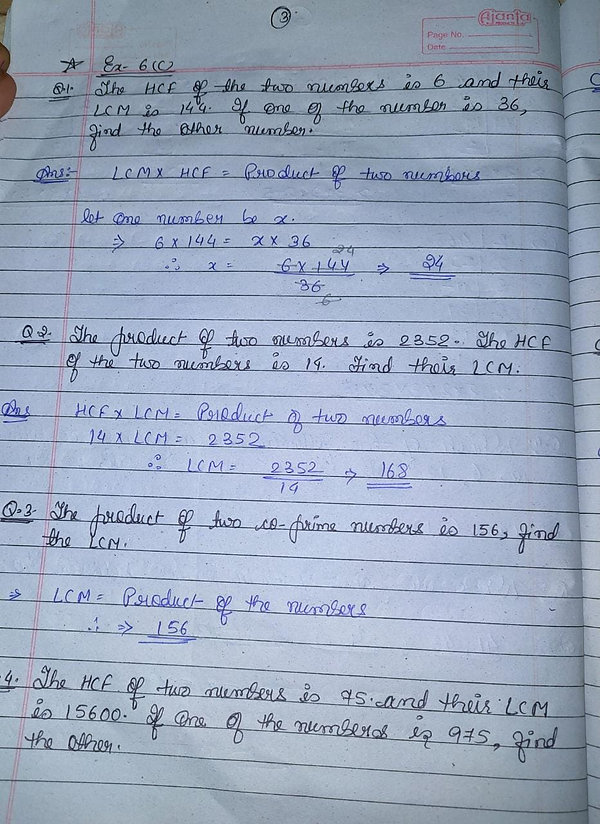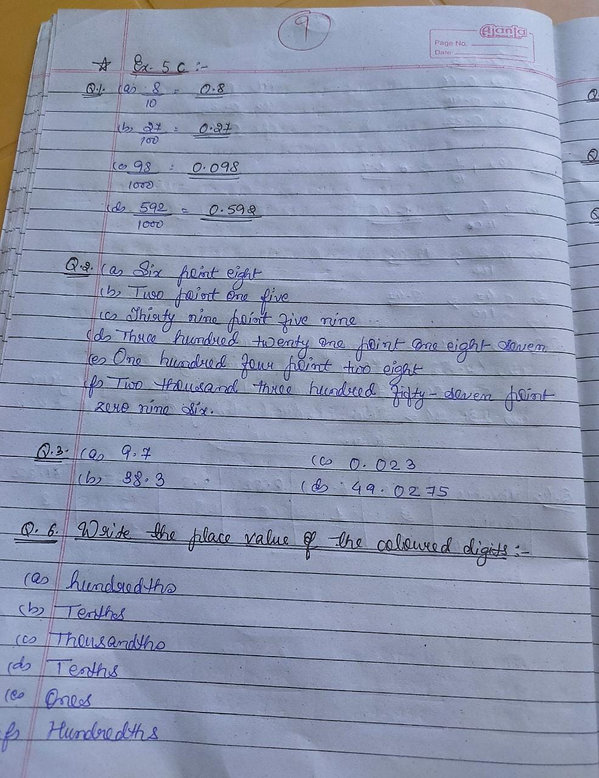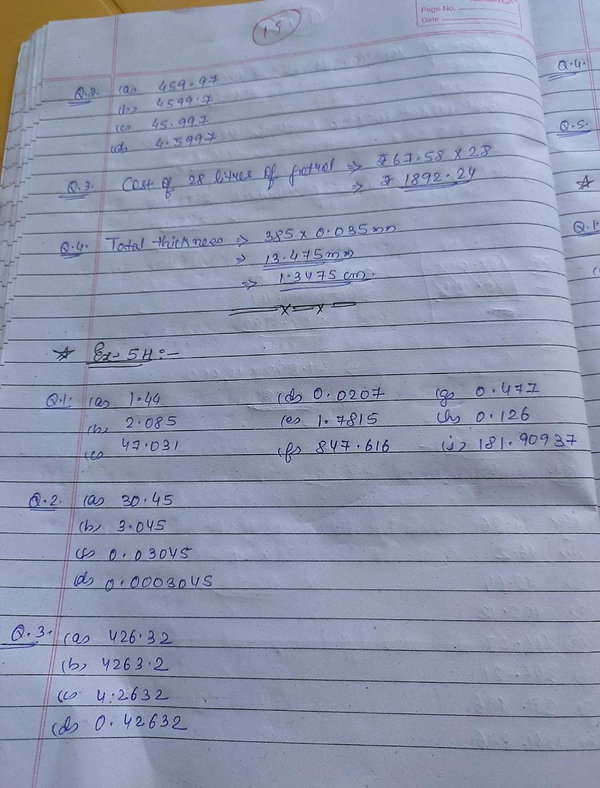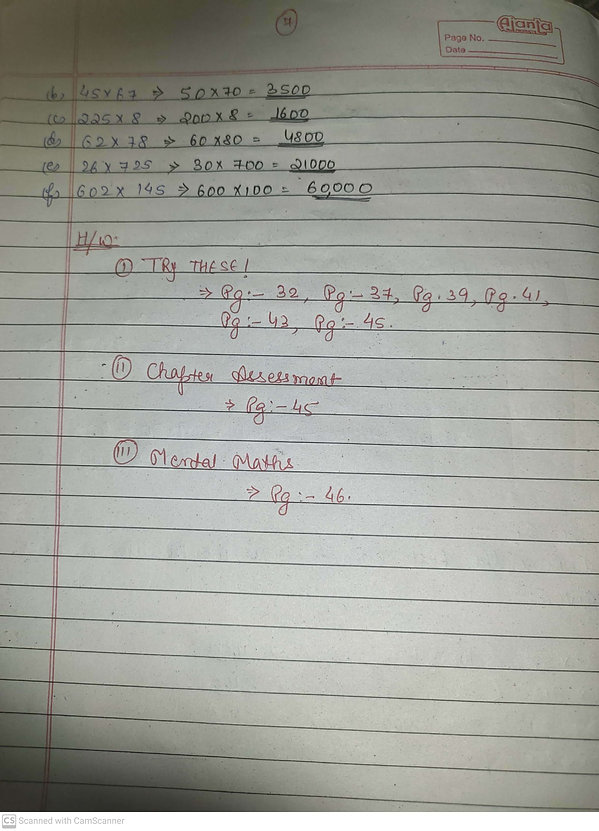Ch-9
Symmetry and Nets of Solids.

•    Reflection means seeing the image of something in a mirror.
•    A line of symmetry is a line that cuts a shape exactly in half.
•    This means that if you were to fold the shape along the line, both halves would match exactly. Equally, if you were to place a mirror along the line, the shape would remain unchanged.
•    Reflection symmetry is when a shape or pattern is reflected in a line of symmetry / a mirror line. The reflected shape will be exactly the same as the original, the same distance from the mirror line and the same size.
•    Rotation in symmetry- A shape has rotational symmetry when it can be rotated and it still looks the same.
•    The order of rotational symmetry of a shape is the number of times it can be rotated around a full circle and still look the same
•     Three things are needed to describe a rotation that are:- 1) Direction of Rotation   2) Angle of Rotation   3) Centre of Rotation.
•    Center of rotation = For a figure or object that has rotational symmetry, the fixed point around which the rotation occurs is called the centre of rotation. Example: the centre of rotation of a windmill in the centre of the windmill from which its blades originate.
•    Angle of Rotation =For a figure or object that has rotational symmetry, the angle of turning during rotation is called the angle of rotation. Example: when a square is rotated by 90 degrees, it appears the same after rotation. So, the angle of rotation for a square is 90 degrees.
•    Direction of Rotation = Rotation may be clockwise or anticlockwise.
•     If, after a rotation, an object looks exactly the same, we say that it has a rotational symmetry.
•    In a complete turn (of 360°), the number of times an object looks exactly the same is called the order of rotational symmetry. The order of symmetry of a square, for example, is 4 while, for an equilateral triangle, it is 3.
•    An object has rotational symmetry if there is a centre of point which if the object is rotated or turned a certain number if degrees and the object still looks the same.
•    Order of Rotational Symmetry= The number of positions in which a figure can be rotated and still appears exactly as it did before the rotation, is called the order of symmetry.
•    Full turn=360°  ; ½ turn=180°   ; ¼ turn=90°
•    Order of rotational symmetry of Square is 4.
•    Order of rotational symmetry of Rectangle is 4.
•    Order of Rotational symmetry of an equilateral triangle is 3.
•    A circle have infinite order of rotational symmetry as a circle will always fit into its original regardless of how many times it is rotated.
•    Plane of symmetry=  a plane or a surface l that divides the solids into two parts that are mirror images of each other or identical halves.
•    The figure has two lines of symmetry: the horizontal line of symmetry cuts the figure into a top and bottom that are mirror images of each other; the vertical line of symmetry cuts the figure into a left and right that are mirror images of each other.
•    A cone and a sphere have infinite number of planes of symmetry.
•    A net can be folded up to make a 3D shape.
•    There may be several possible nets for one 3D shape.
•    The net of a cube and a square based pyramid.

*WRITE PG. NO. 147 ALSO. SAME AS GIVEN IJ BOOK ALONG WITH DIAGRAMS.